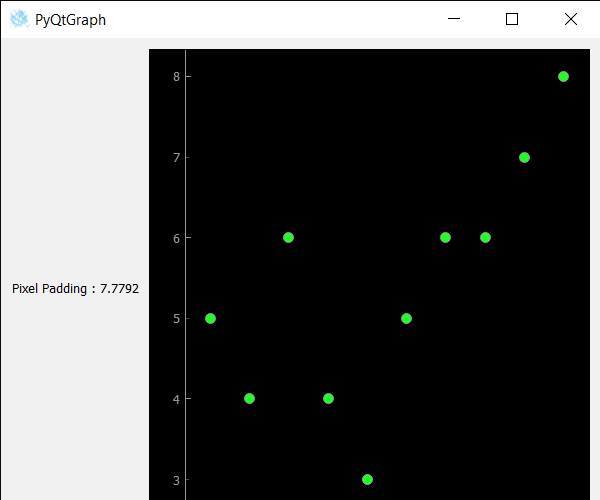GeeksforGeeks App
Open AppBrowser
Continue

# PyQtGraph – Getting Pixel Padding of Spots in Scatter Plot Graph

In this article, we will see how we can get the pixel padding scatter plot graph in the PyQtGraph module. PyQtGraph is a graphics and user interface library for Python that provides functionality commonly required in designing and science applications. Its primary goals are to provide fast, interactive graphics for displaying data (plots, video, etc.). A scatter plot (aka scatter chart, scatter graph) uses dots to represent values for two different numeric variables. It is a type of plot or mathematical diagram using Cartesian coordinates to display values for typically two variables for a set of data. The position of each dot on the horizontal and vertical axis indicates values for an individual data point. Pixel padding is the padding added at the end of the spots which are present in the scatter plot graph.

We can create a plot window and create a scatter plot graph on it with the help of commands given below.

```# creating a pyqtgraph plot window
plt = pg.plot()

# creating a scatter plot graph of size = 10
scatter = pg.ScatterPlotItem(size=10)```

In order to do this we use pixelPadding method with the scatter plot graph object
Argument : It takes no argument
Return : It returns float value

Below is the implementation.

## Python3

 `# importing Qt widgets``from` `PyQt5.QtWidgets ``import` `*` `# importing system``import` `sys` `# importing numpy as np``import` `numpy as np` `# importing pyqtgraph as pg``import` `pyqtgraph as pg``from` `PyQt5.QtGui ``import` `*``from` `PyQt5.QtCore ``import` `*`  `class` `Window(QMainWindow):` `    ``def` `__init__(``self``):``        ``super``().__init__()` `        ``# setting title``        ``self``.setWindowTitle(``"PyQtGraph"``)` `        ``# setting geometry``        ``self``.setGeometry(``100``, ``100``, ``600``, ``500``)` `        ``# icon``        ``icon ``=` `QIcon(``"skin.png"``)` `        ``# setting icon to the window``        ``self``.setWindowIcon(icon)` `        ``# calling method``        ``self``.UiComponents()` `        ``# showing all the widgets``        ``self``.show()` `    ``# method for components``    ``def` `UiComponents(``self``):` `        ``# creating a widget object``        ``widget ``=` `QWidget()` `        ``# creating a label``        ``label ``=` `QLabel(``"Geeksforgeeks Scatter Plot"``)` `        ``# making label do word wrap``        ``label.setWordWrap(``True``)` `        ``# creating a plot window``        ``plot ``=` `pg.plot()` `        ``# number of points``        ``n ``=` `300` `        ``# creating a scatter plot item``        ``# of size = 10``        ``# using brush to enlarge the of green color``        ``scatter ``=` `pg.ScatterPlotItem(``            ``size``=``10``, brush``=``pg.mkBrush(``30``, ``255``, ``35``, ``255``))` `        ``# data for x-axis``        ``x_data ``=` `[``1``, ``2``, ``3``, ``4``, ``5``, ``6``, ``7``, ``8``, ``9``, ``10``]` `        ``# data for y-axis``        ``y_data ``=` `[``5``, ``4``, ``6``, ``4``, ``3``, ``5``, ``6``, ``6``, ``7``, ``8``]` `        ``# adding spots to the scatter plot``        ``scatter.addPoints(x_data, y_data)` `        ``# add item to plot window``        ``# adding scatter plot item to the plot window``        ``plot.addItem(scatter)` `        ``# Creating a grid layout``        ``layout ``=` `QGridLayout()` `        ``# minimum width value of the label``        ``label.setMinimumWidth(``130``)` `        ``# setting this layout to the widget``        ``widget.setLayout(layout)` `        ``# adding label in the layout``        ``layout.addWidget(label, ``1``, ``0``)` `        ``# plot window goes on right side, spanning 3 rows``        ``layout.addWidget(plot, ``0``, ``1``, ``3``, ``1``)` `        ``# setting this widget as central widget of the main window``        ``self``.setCentralWidget(widget)` `        ``# getting pixel padding of the scatter plot``        ``value ``=` `scatter.pixelPadding()` `        ``# setting text to the value``        ``label.setText(``"Pixel Padding : "` `+` `str``(value))`  `# create pyqt5 app``App ``=` `QApplication(sys.argv)` `# create the instance of our Window``window ``=` `Window()` `# start the app``sys.exit(App.``exec``())`

Output:My Personal Notes arrow_drop_up